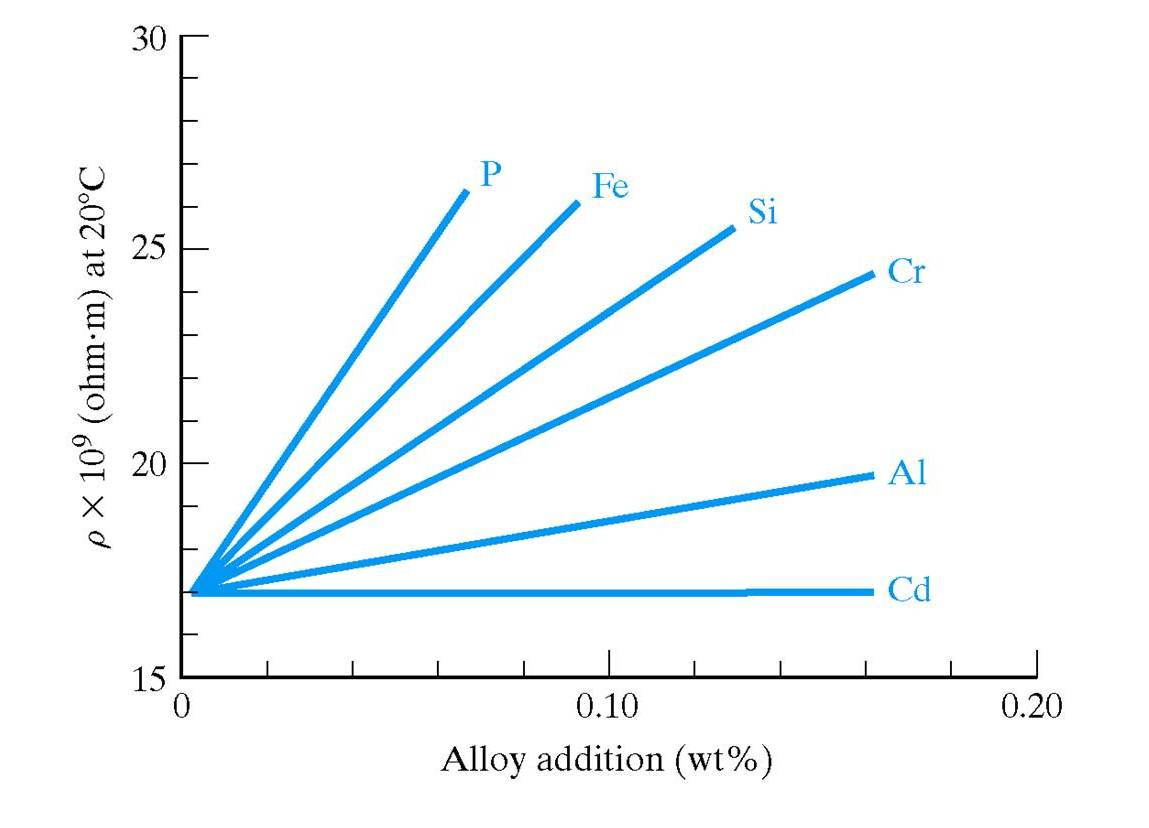How Does Adding Impurities Affect the Conductivity of a Conductor?
Let’s Consider the effect of adding impurities on the conductivity of a conductor.

Again, Let's look at the factors that go into conductivity and consider how each of these are affected:
sigma = n q m
• First consider what will happen to n as impurities are added. Nothing! So number of charge carriers will not change for a conductor with an increase inimpurities.
• Now consider q. As impurities are added, the charge on each carrier will not change.

• Finally, what happens to the mobility? If you consider that impurities will distort the crystal lattice, hence impeding the drift velocity, then you will see that the mobility will decrease. This is similar to the argument for the fact that mobility will decrease with an increase in temperature.

Conclusion:
The electrical conductivity of a conductor will decrease with an increase in impurities!

Again the relationship is not linear, however, if we consider the resistivity, which is the reciprocal of conductivity, we do get a linear relationship:
rho = rho0[1 + beta x]
where
• x is the % of impurities
• rho0 is the resistivity of the material for 0% impurities
• beta is a constant for a given system

Here is a plot showing the variation of electrical resistivity with composition (impurity additions) for various copper alloys. The data is fixed at room temperature (20oC).previous page back to ENGN 45 class page next page